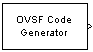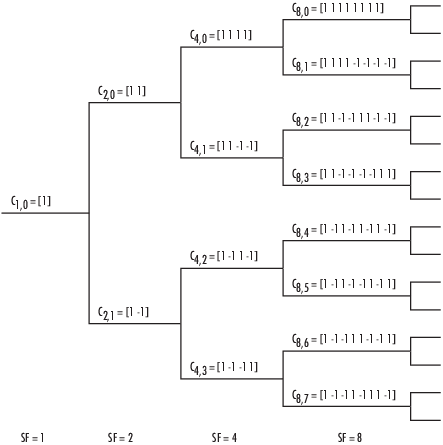# OVSF Code Generator

Generate orthogonal variable spreading factor (OVSF) code from set of orthogonal codes

## Library

•## Description

The OVSF Code Generator block generates an OVSF code from a set of orthogonal codes. OVSF codes were first introduced for 3G communication systems. OVSF codes are primarily used to preserve orthogonality between different channels in a communication system.

OVSF codes are defined as the rows of an N-by-N matrix, CN, which is defined recursively as follows. First, define C1 = . Next, assume that CN is defined and let CN(k) denote the kth row of CN. Define C2N by

`${C}_{2N}=\left[\begin{array}{cc}{C}_{N}\left(0\right)& {C}_{N}\left(0\right)\\ {C}_{N}\left(0\right)& -{C}_{N}\left(0\right)\\ {C}_{N}\left(1\right)& {C}_{N}\left(1\right)\\ {C}_{N}\left(1\right)& -{C}_{N}\left(1\right)\\ ...& ...\\ {C}_{N}\left(N-1\right)& {C}_{N}\left(N-1\right)\\ {C}_{N}\left(N-1\right)& -{C}_{N}\left(N-1\right)\end{array}\right]$`

Note that CN is only defined for N a power of 2. It follows by induction that the rows of CN are orthogonal.

The OVSF codes can also be defined recursively by a tree structure, as shown in the following figure.If [C] is a code length 2r at depth r in the tree, where the root has depth 0, the two branches leading out of C are labeled by the sequences [C C] and [C -C], which have length 2r+1. The codes at depth r in the tree are the rows of the matrix CN, where N = 2r.

Note that two OVSF codes are orthogonal if and only if neither code lies on the path from the other code to the root. Since codes assigned to different users in the same cell must be orthogonal, this restricts the number of available codes for a given cell. For example, if the code C41 in the tree is assigned to a user, the codes C10, C20, C82, C83, and so on, cannot be assigned to any other user in the same cell.

### Block Parameters

You specify the code the OVSF Code Generator block outputs by two parameters in the block's dialog: the Spreading factor, which is the length of the code, and the Code index, which must be an integer in the range [0, 1, ... , N - 1], where N is the spreading factor. If the code appears at depth r in the preceding tree, the Spreading factor is 2r. The Code index specifies how far down the column of the tree at depth r the code appears, counting from 0 to N - 1. For CN, k in the preceding diagram, N is the Spreading factor and k is the Code index.

You can recover the code from the Spreading factor and the Code index as follows. Convert the Code index to the corresponding binary number, and then add 0s to the left, if necessary, so that the resulting binary sequence x1 x2 ... xr has length r, where r is the logarithm base 2 of the Spreading factor. This sequence describes the path from the root to the code. The path takes the upper branch from the code at depth i if xi = 0, and the lower branch if xi = 1.

To reconstruct the code, recursively define a sequence of codes Ci for as follows. Let C0 be the root . Assuming that Ci has been defined, for i < r, define Ci+1 by

The code CN has the specified Spreading factor and Code index.

For example, to find the code with Spreading factor `16` and Code index `6`, do the following:

1. Convert 6 to the binary number 110.

2. Add one 0 to the left to obtain 0110, which has length 4 = log2 16.

3. Construct the sequences Ci according to the following table.

ixiCi
0 C0 = 
1 0 C1 = C0 C0 =  
2 1 C2 = C1 -C1 = [1 1] [-1 -1]
3 1 C3 = C2 -C2 = [1 1 -1 -1] [-1 -1 1 1]
4 0 C4 = C3 C3 = [1 1 -1 -1 -1 -1 1 1] [1 1 -1 -1 -1 -1 1 1]

The code C4 has Spreading factor `16` and Code index `6`.

## Parameters

Positive integer that is a power of 2, specifying the length of the code.

Code index

Integer in the range [0, 1, ... , N - 1] specifying the code, where N is the Spreading factor.

Sample time

Positive scalars specify the time in seconds between each sample of the output signal. If you set the Sample time to `-1`, the output signal inherits the sample time from downstream. For information on the relationship between the Sample time and Samples per frame parameters, see Sample Timing.

Samples per frame

Samples per frame, specified as a positive integer indicating the number of samples per frame in one channel of the output data. For information on the relationship between Sample time and Samples per frame, see Sample Timing.

Output data type

The output type of the block can be specified as an `int8` or `double`. By default, the block sets this to `double`.

Simulate using

Select the simulation mode.

`Code generation`

On the first model run, simulate and generate code. If the structure of the block does not change, subsequent model runs do not regenerate the code.

If the simulation mode is ```Code generation```, System objects corresponding to the blocks accept a maximum of nine inputs.

`Interpreted execution`

Simulate model without generating code. This option results in faster start times but can slow subsequent simulation performance.

expand all

## Version History

Introduced before R2006a

expand all

Behavior changed in R2020a